/

### Function Description

Returns the price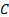of a European call option assuming that the (generalised) Black-Scholes (i.e. Garman-Kohlhagen) pricing formula applies.

If the current price of the underlying is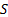, the strike (i.e. exercise) price of the option is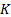, the continuously compounded rate of interest is, the continuously compounded annualised dividend yield (assumed continuous not discrete) is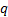, time now (in years) is, time at expiry is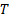and the (annualised) implied volatility is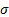then the price of such an option is given by: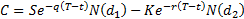where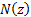is the cumulative unit normal distribution function (see MnCumulativeNormal), i.e.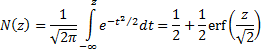and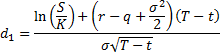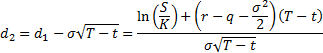Contents | Prev | Next